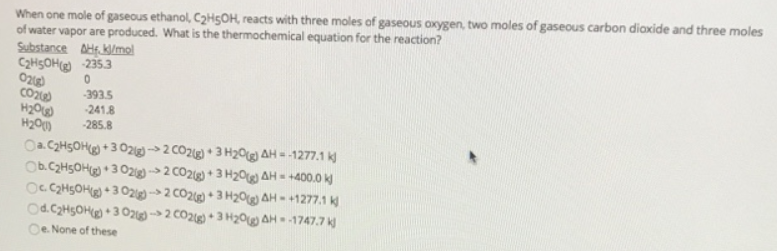# Problem: When one mole of gasecus ethanol, C2H5OH, reacts with three moles of gaseous oxygen, two moles of gaseous carbon diaxide and three moles of water vapor are produced. What is the thermochemical equation for the reaction? a. C2H5OH (g) + 3O2 (g) → 2CO2 (g) + 3H2O (g) ΔH = -1277.1 kJb. C2H5OH (g) + 3O2 (g) → 2CO2 (g) + 3H2O (g) ΔH = +400.0 kJc. C2H5OH (g) + 3O2 (g) → 2CO2 (g) + 3H2O (g) ΔH = +1277.1 kJd. C2H5OH (g) + 3O2 (g) → 2CO2 (g) + 3H2O (g) ΔH = -1747.7 kJe. None of these

###### FREE Expert Solution
82% (306 ratings)###### Problem Details

When one mole of gasecus ethanol, C2H5OH, reacts with three moles of gaseous oxygen, two moles of gaseous carbon diaxide and three moles of water vapor are produced. What is the thermochemical equation for the reaction?

a. C2H5OH (g) + 3O2 (g) → 2CO2 (g) + 3H2O (g) ΔH = -1277.1 kJ

b. C2H5OH (g) + 3O2 (g) → 2CO2 (g) + 3H2O (g) ΔH = +400.0 kJ

c. C2H5OH (g) + 3O2 (g) → 2CO2 (g) + 3H2O (g) ΔH = +1277.1 kJ

d. C2H5OH (g) + 3O2 (g) → 2CO2 (g) + 3H2O (g) ΔH = -1747.7 kJ

e. None of these